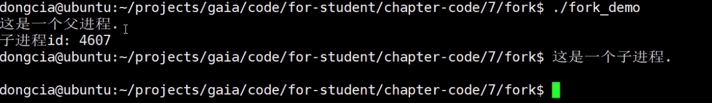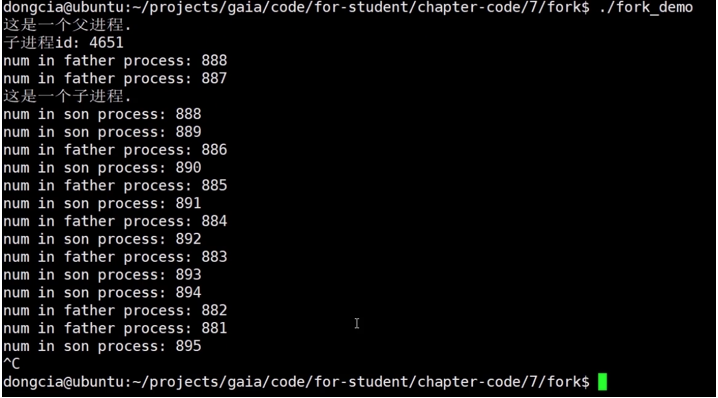# 计算机操作系统基础 (十五)--- 使用 fork 系统调用创建进程## 使用 fork 系统调用创建进程

• fork 系统调用是用于创建进程

• fork 创建的进程初始化状态是和父进程一样的(进程有进程空间、内存、内存态等)

• 系统会为 fork 的进程分配新的资源(包括内存资源、CPU 资源等)

• fork 系统调用无参数

• fork 会返回两次，分别返回子进程 id 和 0(第一次是由父进程返回的，第二次由子进程所返回的，因此返回了两次)

• 返回子进程 id 的是父进程，返回 0 的是子进程

#include<iostream>#include<cstring>#include<stdio.h>#include<unistd.h>using namespace std;int main(){    pid_t pid;    pid = fork();    if(pid == 0) {        cout << "这是一个子进程" << endl;    }    else if(pid > 0) {        cout << "这是一个父进程" << endl;        cout << "子进程id：" << pid << endl;    }    else if(pid < 0 ){        cout << "创建进程失败" << endl;    }    return 0;}#include<iostream>#include<cstring>#include<stdio.h>#include<unistd.h>using namespace std;int main(){    pid_t pid;    int num = 888;    pid = fork();    if(pid == 0) {        cout << "这是一个子进程" << endl;        cout << "num in son process："<< num << endl;        while(true) {            num+=1;            cout << "num in son process："<< num << endl;            sleep(1);        }    }    else if(pid > 0) {        cout << "这是一个父进程" << endl;        cout << "子进程id：" << pid << endl;        cout << "num in father process："<< num << endl;        while(true) {            num-=1;            cout << "num in father process："<< num << endl;            sleep(1);        }    }    else if(pid < 0 ){        cout << "创建进程失败" << endl;    }    return 0;}## 评论